# Complex Numbers: Multiplication and Rotation

Having looked at the idea of complex numbers and how to perform basic operations on them, we are ready for one of the most important features for applications: their relationship to rotation. We’ll see this first in describing complex numbers by a length and an angle (polar form), then by discovering the meaning of multiplication and of powers in this two-dimensional world of numbers (DeMoivre’s Theorem).

## Polar form: angles and distances

Polar Representation of Complex Numbers

A) The polar representation of a complex number z=a+ib is z = r[cos(x) + i sin(x)]. Express the quantities r and x in terms of a and b.

B) Represent the quantities r and x geometrically.

C) Give two examples of complex numbers and find their corresponding polar representation.

D) What is/are the value(s) of x for a number that is purely real.

E) What is/are the value(s) of x for a number that has real part equal to zero.

It looks like Christie is just being introduced to the ideas of polar representation of complex numbers. I answered, mostly explaining what she was being asked to do:

Hi, Christie.

What you are calling x, more often denoted by the Greek letter theta, is the angle from the positive x axis to the number z; r is the distance from the origin to z: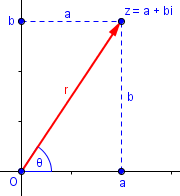This answers the second question, the geometrical interpretation.

I’ve used $$\theta$$ (theta) in the picture, but continue to use Christie’s “x” in the answer; we commonly use x for the real part and y for the imaginary part, so using x also for an angle can be confusing. The representation using r and $$\theta$$ is called polar form, equivalent to polar coordinates; the representation using $$a+bi$$ is, in contrast, called rectangular form.

The angle $$\theta$$ is often called the argument of the complex number, $$\arg(z)$$, or (sometimes) the phase; the distance $$r$$ is called the absolute value, magnitude, or modulus, $$|z|$$. (The terms argument and modulus, are used in too many other ways in math, so I find them confusing; but we have to use them because many students do. In this case, we aren’t given any names for them, so I didn’t worry about that!)

Christie was told how to find a and b from r and $$\theta$$ (“x“), as part of the provided formula, so I explained that part:

You can use trigonometry to show that, as indicated in the problem, the real part a will be r cos(x) and the imaginary part b will be r sin(x). Similarly, you can find r and x from the picture, using the Pythagorean theorem and the definition of a trig function.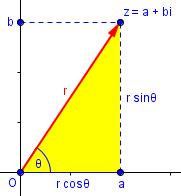These relationships are true because $$\frac{a}{r}=\cos(\theta)$$ and $$\frac{b}{r}=\sin(\theta)$$.

This is what was given in the problem: $$z=a+bi=(r\cos\theta)+(r\sin\theta)i=r(\cos\theta+i\sin\theta)$$ (Note that we often write i before any expression that might otherwise be misread.)

But she was also asked to reverse this, finding $$r$$ and $$\theta$$ in terms of a and b. Since r is the distance from the origin to the point, which as we saw last time is its absolute value, the Pythagorean theorem tells us that $$r=|z|=\sqrt{a^2+b^2}.$$ For $$\theta$$, the angle from the positive x-axis to the point, we can see that  $$\tan\theta=\frac{b}{a}.$$

But actually expressing $$\theta$$ itself in terms of a and b is tricky. I only suggested the general ideas, expecting further questions when Christie thought thought about it more. The issue here is that we can’t just say $$\theta=\tan^{-1}\frac{b}{a}$$ because that would always give angles in the interval $$-\frac{\pi}{2}<\theta<\frac{\pi}{2}$$, and it doesn’t work for pure imaginary numbers where $$a=0$$, or in other quadrants. In general, it can be any angle with the correct terminal ray, but sometimes you are expected to give the “principal value” of the argument, in some particular interval, commonly $$-\pi<\theta\le\pi$$. So I couldn’t be sure what answer is required in her specific context. One way or another, you need to use various tools of trigonometry to find the angle given the tangent together with the quadrant, not a simple formula. (In computer programming, we define a special function atan2(y, x) that gives an appropriate angle in whatever quadrant is needed, but this is not generally taught in algebra or trigonometry. Rather, we teach a procedure I’ll be illustrating here.)

You can pick any numbers you want for part (c); try 1+i and 1-i for starters, and then try one where x is 60 degrees.

Here I’m suggesting two ways to make up examples. The first is to start with the rectangular form and find the polar form, which is what the problem explicitly asks for; my suggestions are among the few for which the angle you find will be “nice”: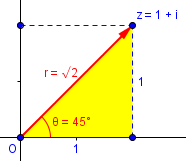Notice that here we meet the question of what interval to use for $$\theta$$. Although what I showed here is a valid answer, it is common to use a “principal value” for the angle, in the interval $$(-\pi,\pi]$$, so our answer would be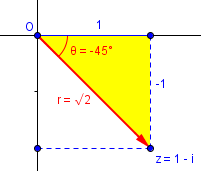In any case, we would find this angle by finding the reference angle, $$\arctan\left(\frac{|-1|}{|1|}\right)=\frac{\pi}{4}$$ or 45°, and adjusting for the quadrant (quadrant IV because $$x>0,y<0$$).

My second suggestion was to start with a number in polar form, e.g. $$r=2,\theta=60^\circ$$: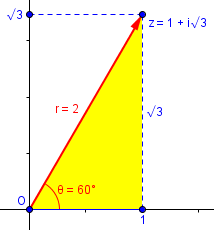Here $$z=r\cos\theta+ir\sin\theta=2\cos60^\circ+2i\sin60^\circ=1+i\sqrt{3}\approx1+1.732i.$$ This is considerably easier to do.

The last two parts are about special cases:

A purely real number, with b=0, will lie on the x-axis (positive or negative). What will the angle x be in my picture? (There are two answers.)

If we have a positive real number, the angle will be $$0^\circ$$: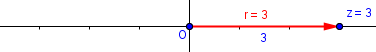If it is negative, the angle is $$180^\circ$$: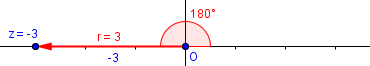A purely imaginary number, with a=0, will lie on the y-axis. What will the angle be?

Again, there are two possibilities (and more if we make different choices for the interval).

Positive multiple of i (we can’t call this a positive imaginary number, because positive numbers are real!):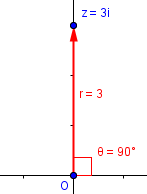Negative multiple of i: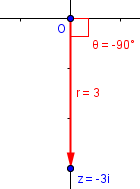## Polar form in “cis” notation

Next, a question from 1996:

Polar to Rectangular Conversions

How do I convert "8 cis 30" into rectangular coordinates?  I am totally lost.

The expression "8 cis 30" is a way of writing the complex number "8*(cos(30) + i*sin(30))" which  has Real part 8*cos(30) and has Imaginary part 8*sin(30).  Usually the system of complex numbers is thought of as a plane, similar to the usual Cartesian plane that consists of pairs (X,Y) of real numbers.  In this interpretation, your polar-type expression "8 cis 30" converts to a rectangular ordered pair (8*cos(30) , 8*sin(30)).  The sine of 30 degrees is one half, and the cosine of 30 degrees is one half of the square root of three, so this ordered pair is (4*sqrt(3), 4)

I hope this is the information you needed.

This notation was introduced as a shorthand, to avoid having to write this long expression too often. Where we would have written $$\underline{\text{c}}\text{os}\,\theta + \underline{i}\underline{\text{s}}\text{in}\,\theta$$, we write $$\underline{\text{cis}}\,\theta$$.

Expanding the abbreviation, $$8\text{ cis}\,30^\circ$$ becomes $$8(\cos30^\circ + i\sin30^\circ)=8\cos30^\circ + 8\sin30^\circ i=8\cdot\frac{\sqrt{3}}{2} + 8\cdot\frac{1}{2} i=4\sqrt{3}+4i$$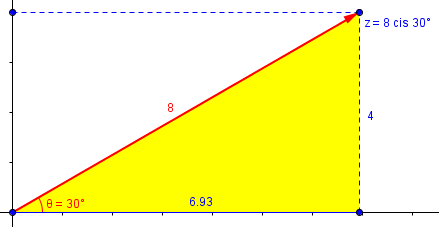This notation is only needed between when you first learn polar form and when you know enough calculus that a more powerful notation, $$re^{i\theta}$$, makes sense. Another notation that is used in some applications, such as electronics, is $$r\angle\theta$$.

## Multiplying and dividing in polar form

Now it’s time for some operations. This is from 1999:

Polar Number Multiplication and Division

I understand that when two polar numbers are multiplied, the real parts multiply and the angles add. I also understand that when two polar numbers are divided, the real parts divide and the angles subtract. However, I do not understand how these rules work. Why is it that when I multiply or divide polar numbers, the angles add or subtract? I tried to convert the polar numbers into complex numbers and then FOIL (First Outer Inner Last) them out. Then I took the complex answer and converted it back to polar. This seemed to show me that two arctangents produce a third arctangent.

For multiplication of polar numbers:

arctan(imag1/real1) + arctan(imag2/real2) = yet another arctangent

For division of polar numbers:

arctan(imag1/real1) - arctan(imag2/real2) = yet another arctangent

This did not seem to prove anything. Is there a proof that will show me the validity of these two polar rules? Do I have the right idea with the arctangent approach? I think I am missing something.

Thank you for your interest and help.

The idea here is that the product of complex numbers has as its absolute value the product of the absolute values, and as its angle the sum of the angles: $$(r_1\text{ cis}(\theta_1))(r_2\text{ cis}(\theta_2))=(r_1r_2)\text{ cis}(\theta_1+\theta_2)$$

(Matt erred in saying “real parts” instead of “absolute values”, but probably meant the right thing!)

But to him this is just a rule, and rules taught without reasons can be confusing. What does it mean, and why in the world would you believe such a claim? He tried to work that out himself, and perhaps came close.

### Proof 1

On the contrary, this proved exactly what you wanted, since

arctan(imag1/real1) = angle1
arctan(imag2/real2) = angle2

yet another,

arctan = angle3

then you have proved that, for multiplication,

angle1 + angle2 = angle3

which is just as it should be.

I’m not sure what Matt might have actually done, but let’s fill in the details!

We’re given a product $$(a+bi)(c+di)=(ac-bd)+(ad+bc)i.$$ Here I’m using single letters rather than Matt’s $$(real_1+imag_1i)(real_2+imag_2i)$$, and the angles for the two factors are (ignoring quadrants) $$\theta_1=\arctan\frac{b}{a},\theta_2=\arctan\frac{d}{c}.$$

Possibly he used the arctangent addition formula, $$\arctan(u)+\arctan(v)=\arctan\frac{u+v}{1-uv},$$ which is a restatement of the tangent addition formula (see below), and may give an answer that is off by a multiple of π. Applying this to what we know, we get $$\arctan\frac{b}{a}+\arctan\frac{d}{c}=\arctan\frac{\frac{b}{a}+\frac{d}{c}}{1-\frac{b}{a}\frac{d}{c}}=\arctan\frac{bc+ad}{ac-bd},$$ which is the angle for the product as already found. The fact that we didn’t properly deal with the quadrant makes this feel a little incomplete, but it does basically show what we wanted.

We could instead use the tangent addition formula directly, avoiding the awkwardness of the inverse tangents, to find the tangent of the sum of the angles: $$\tan(\theta_1+\theta_2)=\frac{\tan\theta_1+\tan\theta_2}{1-\tan\theta_1\tan\theta_2}.$$ Just as before, this gives us $$\tan(\theta_1+\theta_2)=\frac{\frac{b}{a}+\frac{d}{c}}{1-\frac{b}{a}\frac{d}{c}}=\frac{bc+ad}{cd-ab}.$$ But this is in fact the angle for the product $$(ac-bd)+(ad+bc)i$$.

This way, we never had to use actual angles, just the tangents, so quadrants didn’t matter.

### Proof 2

Doctor Schwa offered a slightly different approach, avoiding tangents:

Another way to do the proof is to write the polar numbers like this:

r1 (cos theta1 + i sin theta1) * r2 (cos theta2 + i sin theta2)

Then after foiling, you can use some trigonometric identities to show that the answer is

r1*r2 (cos (theta1 + theta2) + i sin (theta1 + theta2)),

which proves what you needed.

Here we’re working entirely in polar form rather than rectangular form, and we’ll be using sines and cosines instead of tangents. To evaluate the product $$(r_1\text{ cis}(\theta_1))(r_2\text{ cis}(\theta_2))=r_1(\cos\theta_1+i\sin\theta_1)\times r_2(\cos\theta_2+i\sin\theta_2),$$ we first distribute to get $$r_1r_2(\cos\theta_1+i\sin\theta_1)(\cos\theta_2+i\sin\theta_2)\\=r_1r_2(\cos\theta_1\cos\theta_2+\cos\theta_1i\sin\theta_2+i\sin\theta_1\cos\theta_2+i\sin\theta_1i\sin\theta_2)\\=r_1r_2((\cos\theta_1\cos\theta_2-\sin\theta_1\sin\theta_2)+(\cos\theta_1\sin\theta_2+\sin\theta_1\cos\theta_2)i).$$

But angle-sum identities for cosine and sine tell us that $$\cos(\theta_1+\theta_2)=\cos\theta_1\cos\theta_2-\sin\theta_1\sin\theta_2$$ $$\sin(\theta_1+\theta_2)=\cos\theta_1\sin\theta_2+\sin\theta_1\cos\theta_2,$$ so we can rewrite the product as $$r_1r_2(\cos(\theta_1+\theta_2)+i\sin(\theta_1+\theta_2))=(r_1r_2)\text{ cis}(\theta_1+\theta_2).$$ And that’s the goal.

### An example

Let’s look at an example. I’ll multiply $$(1-i)(-1+i\sqrt{3})=(\sqrt{3}-1)+(\sqrt{3}+1)i,$$ which in polar form is $$\sqrt{2}\text{ cis}(-45^\circ)\times2\text{ cis}(120^\circ)$$; the product in polar form is $$2\sqrt{2}\text{ cis}(75^\circ)$$. The graph agrees (blue times red equals green):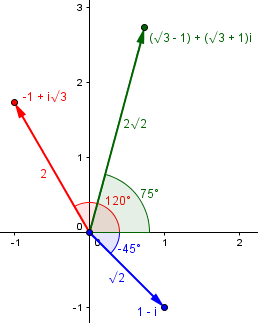So what does multiplication of complex numbers do? It multiplies their absolute values (moduli) and adds their angles (arguments).

Viewed differently, multiplying $${\color{Blue}{\sqrt{2}\text{ cis}(-45^\circ)}}$$ by $${\color{Red}{2\text{ cis}(120^\circ)}}$$, stretches it by a factor of 2 and rotates it by 120° to $${\color{DarkGreen}{2\sqrt{2}\text{ cis}(75^\circ)}}$$: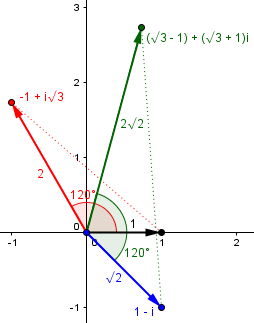Observe the similar triangles, with the same angle, and proportional sides. This is what multiplication is.

## Another proof and example

Another question from 1999:

Why Multiply Two Complex Numbers?

Why do we multiply two complex numbers?  What does that give us, and how do you graph it?

(8 cis 70) * (2 cis 34) = (16 cis 104)

How do you get that?  What is it used for?

Thanks.

We can see that he has multiplied the moduli and added the arguments. He knows what to do, but wonders why this is multiplication.

### Proof 3

I answered, giving essentially Doctor Schwa’s proof:

The basic idea behind multiplying complex numbers is a simple application of the distributive property:

(a + bi)*(c + di) = ac + adi + bci + bdi^2
= (ac - bd) + (ad + bc)i


(In what follows, I use the same letters with different meanings – shame on me!)

Someone discovered that this fits very neatly with the polar ("cis") representation of the numbers:

(a cis b)*(c cis d) = (a cos(b) + a i sin(b))*(c cos(d) + c i sin(d))
= [ac cos(b) cos(d) - ac sin(b) sin(d)] +
[ac sin(b) cos(d) + ac cos(b) sin(d)]i
= ac cos(b+d) + i ac sin(b+d)
= ac cis (b+d)

So we can directly derive this fact. We’ll be seeing still more next week of how trigonometric facts can be seen in complex number arithmetic.

So this method of multiplication of complex numbers comes directly from the angle-sum identities in trigonometry. This gives us a simple way to see complex multiplication in terms of vectors: multiply the lengths and add the angles. But that does not originate in any vector concept; it is simply the natural result of defining multiplication of complex numbers to follow the same rules as real numbers. Addition of vectors is a natural concept; multiplication applies only to complex numbers.

### The example

Here is Angkit’s problem, in rectangular form:

$$8\text{ cis}(70^\circ)=8\cos(70^\circ)+8i\sin(70^\circ)\approx2.736+7.518i$$

$$2\text{ cis}(34^\circ)=2\cos(34^\circ)+2i\sin(34^\circ)\approx1.658+1.118i$$

$$16\text{ cis}(104^\circ)=16\cos(104^\circ)+16i\sin(104^\circ)\approx-3.871+15.525i.$$

And $$(2.736+7.518i)(1.658+1.118i)\approx-3.869+15.524i.$$

Here are the three points, confirming the angles (again, blue times red equals green):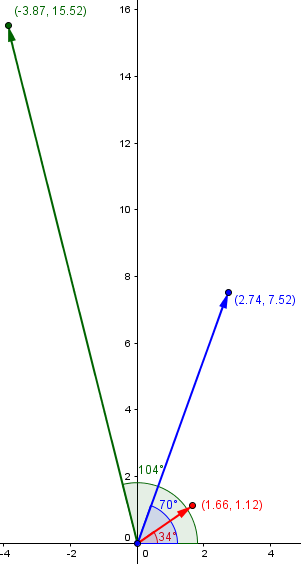Again, just as adding a complex number shifts a point on the complex plane in that direction, multiplying by a complex number rotates a point by that angle, and stretches it by its magnitude. This is what makes complex numbers powerful in applications!

## DeMoivre’s theorem: powers of complex numbers

Now we can move on to powers and roots!

This is from 1998:

Trigonometry and Complex Numbers

Simplify (sqrt 3 - i)^7 into the form a + bi.

We could just multiply 7 copies of this number together; but that takes a lot of work. One way to do it efficiently is to repeatedly square, and then combine those results:

$$z=\sqrt{3}-i\\z^2=(\sqrt{3}-i)(\sqrt{3}-i)=2-2i\sqrt{3}\\z^4=(2-2i\sqrt{3})(2-2i\sqrt{3})=-8-8i\sqrt{3}$$

$$z^3=z\cdot z^2=(\sqrt{3}-i)(2-2i\sqrt{3})=0-8i$$

$$z^7=z^3\cdot z^4=(-8)(-8-8i\sqrt{3})=-64\sqrt{3}+64i$$

We’ll see these graphically later.

Can we do it more easily? Yes!

Hi Eric,

The trick to solving this problem is putting the number into complex polar form, using DeMoivre's Theorem, then transforming the answer back into rectangular form (a + bi).

Here is my suggestion. Locate the point sqrt(3) - i on the complex coordinate plane, then draw a segment from the point to the origin and draw another segment from the point perpendicular to the positive x-axis. Using the Pythagorean Theorem you can determine that the hypotenuse of the triangle you have drawn has length 2. Furthermore, the angle between the hypotenuse and the positive x-axis is 30 degrees in a clockwise direction, so we'll call it -30 degrees.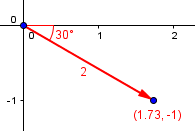Now, polar coordinate form looks like r(cos T + i sin T) where r is the distance from the point to the origin (in this case, 2), and T is the angle that the segment from the point to the origin makes with the positive x-axis. (in this case, -30 degrees).

So, our expression in complex polar form is:

[2(cos(-30) + i sin(-30)]^7

You may already see what’s coming. What will happen to the modulus each time we multiply? It will be multiplied. What will happen to the argument? It will be added to (rotated). For now, we’ll just apply a theorem that is implied by these facts (and will be proved momentarily):

$$(a+bi)^n=(r\text{ cis}(\theta))^n=r^n\text{ cis}(n\theta)$$

According to DeMoivre's Theorem:

[2(cos(-30) + i sin(-30)]^7 = 2^7 [cos((-30)(7)) + i sin((-30)(7))]

We now can evaluate 2^7 = 128 and (-30)(7) = -210.

The result is 128(cos(-210) + i sin(-210)).

Note cos(-210) = -sqrt(3)/2 and sin(-210) = 1/2.

Therefore, the final answer is -64*sqrt(3) + 64i.

An interesting thing to observe in this specific case is that $$z^7=-64z$$. Do you see why? We multiplied 6 times, rotating 30° each time, for a total of 180°, and scaled up by a factor 2 to 6 times, multiplying the length by 64.

Here I have plotted the successive powers of our $$z$$, from the first ($$z$$ itself) to the seventh: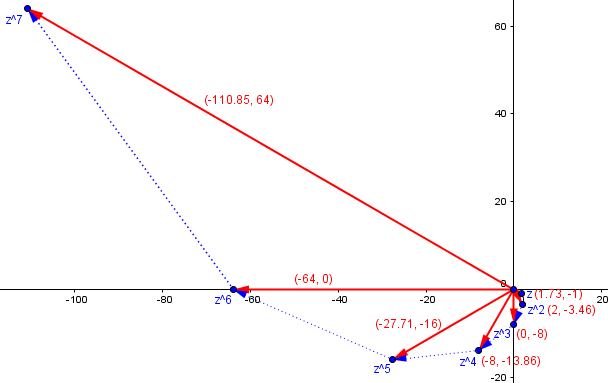## Proving the theorem by induction

Every good theorem deserves a proof, so we’ll end with this question from 1997:

Proof of DeMoivre's Theorem

Could you please help me prove DeMoivre's theorem by mathematical induction?  I figured out the first part of the proof, but I can't prove that assuming that if it's true for n = k, then it's true for n = k+1.

We discussed induction in a series starting here. Evelina has presumably seen that the basis step, proving it true for $$n=1$$ (or, indeed, 0) is true – which is obvious – but needs help with this inductive step.

Evelina,

This depends on the addition formulas for the sine and cosine:

sin(x + y) = sin(x) cos(y) + sin(y) cos(x)

cos(x + y) = cos(x) cos(y) - sin(x) sin(y)

Sound familiar? That’s what we used to prove multiplication. We’ll be doing that repeatedly here.

DeMoivre's theorem states that:

(cos(x) + i sin(x))^n = cos(nx) + i sin(nx)

In its basic form, it is assumed that n is a positive integer; that allows us to use induction.

In your case, you are assuming this holds for n = k and are trying to prove that it holds for n = k + 1, so you are looking at:

(cos(x) + i sin(x))^(k + 1)

This is just:

(cos(x) + i sin(x))^k * (cos(x) + i sin(x))

Using the induction hypothesis, this is:

(cos(kx) + i sin(kx)) ( cos(x) + i sin(x))

Multiplying out and using the fact that i^2 = -1, we get:

cos(kx) cos(x) - sin(kx) sin(x) + i (sin(kx) cos(x) + cos(kx) sin(x))

This is just cos((k+1) x) + i sin((k+1) x) by the addition formulas mentioned above.

I recommend that you study this proof carefully, as it is very typical of induction proofs.

Incidentally, it turns out that this formula applies even when the exponent n is not positive, or even is not an integer. But that requires a different sort of proof. We’ll eventually get to roots of complex numbers; but first, next week, we have to look at a calculus perspective on all this, which unifies the ideas even more.

### 3 thoughts on “Complex Numbers: Multiplication and Rotation”

This site uses Akismet to reduce spam. Learn how your comment data is processed.